The ratio of the relative rise in pressure for adiabatic compression to that for isothermal compression is

(1) $\gamma$

(2) $\frac{1}{\gamma }$

(3) 1-$\gamma$

(4) $\frac{1}{1-\gamma }$

Concept Questions :-

Types of processes
High Yielding Test Series + Question Bank - NEET 2020

Difficulty Level:

A sink, that is the system where heat is rejected, is essential for the conversion of heat into work. From which law the above inference follows?

(1) zeroth

(2) First

(3) Second

(4) Third

Concept Questions :-

Second law of thermodynamics
High Yielding Test Series + Question Bank - NEET 2020

Difficulty Level:

For the indicator diagram given below to select the wrong statement.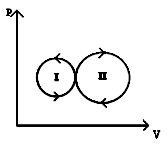1.  Cycle - II is a heat engine cycle

2.   Net work is done on the gas in cycle - I

3.   Work done is positive for the cycle I

4.  Work done is positive for the cycle - II

Concept Questions :-

Work done by gas
High Yielding Test Series + Question Bank - NEET 2020

Difficulty Level:

The variation of pressure versus temperature of an ideal gas is shown in the given diagram. From this diagram, one can conclude that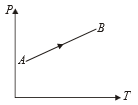1.  Volume increases continuously

2.  Volume decreases continuously

3.  Volume first increases then decreases

4.  Volume first decreases, then increase

Concept Questions :-

Types of processes
High Yielding Test Series + Question Bank - NEET 2020

Difficulty Level:

The variation of density (p) of gas with its absolute temperature (T) at constant pressure is best represented by the graph

1.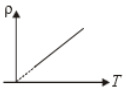2.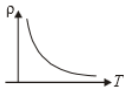3.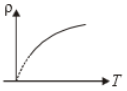4.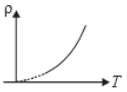Concept Questions :-

Types of processes
High Yielding Test Series + Question Bank - NEET 2020

Difficulty Level:

An ideal gas with adiabatic exponent y is heated at constant pressure and it absorbs Q heat. What fraction of this heat is used to perform external work

1.  $\mathrm{\gamma }$

2.  $\frac{\mathrm{\gamma }}{\mathrm{\gamma }}$

3.

4.

Concept Questions :-

Types of processes
High Yielding Test Series + Question Bank - NEET 2020

Difficulty Level:

A Carnot engine working between 400K and 800K has a work output of 900J per cycle. The amount of heat energy supplied to engine from the source per cycle is

1.  900 J

2.  1800 J

3.  450 J

4.  2700 J

Concept Questions :-

Heat engine and refrigerator
High Yielding Test Series + Question Bank - NEET 2020

Difficulty Level:

Temperature is defined by

1.  First Law of thermodynamics

2.  Second Law of thermodynamics

3.  Third Law of  thermodynamics

4.  Zeroth Law of  thermodynamics

Concept Questions :-

Basic terms
High Yielding Test Series + Question Bank - NEET 2020

Difficulty Level:

The P-V diagram for a thermodynamic system is shown in the figure. The work done by the system during the cyclic process ABCA is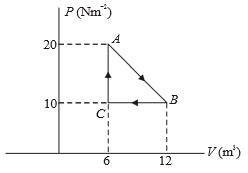1.  Zero

2.  90 J

3.  30 J

4.  60 J

Concept Questions :-

Work done by gas
High Yielding Test Series + Question Bank - NEET 2020

Difficulty Level:

If 32 gm of ${O}_{2}$ at $27°\mathrm{C}$ is mixed with 64 gm of ${\mathrm{O}}_{2}$ at $327°\mathrm{C}$ in an adiabatic vessel, then the final temperature of the mixture will be :

1.  $200°\mathrm{C}$

2.  $227°\mathrm{C}$

3.  $314.5°\mathrm{C}$

4.  $235.5°\mathrm{C}$

Concept Questions :-

Molar Specific heat